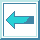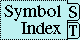#Macro DEFMACRO

Syntax:

defmacro name lambda-list [[declaration* | documentation]] form*

=> name

Arguments and Values:

name---a symbol. lambda-list---a macro lambda list.

declaration---a declare expression; not evaluated.

documentation---a string; not evaluated.

form---a form.

Description:

Defines name as a macro by associating a macro function with that name in the global environment. The macro function is defined in the same lexical environment in which the defmacro form appears.

The parameter variables in lambda-list are bound to destructured portions of the macro call.

The expansion function accepts two arguments, a form and an environment. The expansion function returns a form. The body of the expansion function is specified by forms. Forms are executed in order. The value of the last form executed is returned as the expansion of the macro. The body forms of the expansion function (but not the lambda-list) are implicitly enclosed in a block whose name is name.

The lambda-list conforms to the requirements described in Section 3.4.4 (Macro Lambda Lists).

Documentation is attached as a documentation string to name (as kind function) and to the macro function.

defmacro can be used to redefine a macro or to replace a function definition with a macro definition.

Recursive expansion of the form returned must terminate, including the expansion of other macros which are subforms of other forms returned.

The consequences are undefined if the result of fully macroexpanding a form contains any circular list structure except in literal objects.

If a defmacro form appears as a top level form, the compiler must store the macro definition at compile time, so that occurrences of the macro later on in the file can be expanded correctly. Users must ensure that the body of the macro can be evaluated at compile time if it is referenced within the file being compiled.

Examples:

``` (defmacro mac1 (a b) "Mac1 multiplies and adds"
`(+ ,a (* ,b 3))) =>  MAC1
(mac1 4 5) =>  19
(documentation 'mac1 'function) =>  "Mac1 multiplies and adds"
(defmacro mac2 (&optional (a 2 b) (c 3 d) &rest x) `'(,a ,b ,c ,d ,x)) =>  MAC2
(mac2 6) =>  (6 T 3 NIL NIL)
(mac2 6 3 8) =>  (6 T 3 T (8))
(defmacro mac3 (&whole r a &optional (b 3) &rest x &key c (d a))
`'(,r ,a ,b ,c ,d ,x)) =>  MAC3
(mac3 1 6 :d 8 :c 9 :d 10) =>  ((MAC3 1 6 :D 8 :C 9 :D 10) 1 6 9 8 (:D 8 :C 9 :D 10))
```

The stipulation that an embedded destructuring lambda list is permitted only where ordinary lambda list syntax would permit a parameter name but not a list is made to prevent ambiguity. For example, the following is not valid:

``` (defmacro loser (x &optional (a b &rest c) &rest z)
...)
```
because ordinary lambda list syntax does permit a list following &optional; the list (a b &rest c) would be interpreted as describing an optional parameter named a whose default value is that of the form b, with a supplied-p parameter named &rest (not valid), and an extraneous symbol c in the list (also not valid). An almost correct way to express this is

``` (defmacro loser (x &optional ((a b &rest c)) &rest z)
...)
```
The extra set of parentheses removes the ambiguity. However, the definition is now incorrect because a macro call such as (loser (car pool)) would not provide any argument form for the lambda list (a b &rest c), and so the default value against which to match the lambda list would be nil because no explicit default value was specified. The consequences of this are unspecified since the empty list, nil, does not have forms to satisfy the parameters a and b. The fully correct definition would be either

``` (defmacro loser (x &optional ((a b &rest c) '(nil nil)) &rest z)
...)
```
or

``` (defmacro loser (x &optional ((&optional a b &rest c)) &rest z)
...)
```
These differ slightly: the first requires that if the macro call specifies a explicitly then it must also specify b explicitly, whereas the second does not have this requirement. For example,

``` (loser (car pool) ((+ x 1)))
```
would be a valid call for the second definition but not for the first.

``` (defmacro dm1a (&whole x) `',x)
(macroexpand '(dm1a))  =>  (QUOTE (DM1A))
(macroexpand '(dm1a a)) is an error.

(defmacro dm1b (&whole x a &optional b) `'(,x ,a ,b))
(macroexpand '(dm1b))  is an error.
(macroexpand '(dm1b q))  =>  (QUOTE ((DM1B Q) Q NIL))
(macroexpand '(dm1b q r)) =>  (QUOTE ((DM1B Q R) Q R))
(macroexpand '(dm1b q r s)) is an error.
```

``` (defmacro dm2a (&whole form a b) `'(form ,form a ,a b ,b))
(macroexpand '(dm2a x y)) =>  (QUOTE (FORM (DM2A X Y) A X B Y))
(dm2a x y) =>  (FORM (DM2A X Y) A X B Y)

(defmacro dm2b (&whole form a (&whole b (c . d) &optional (e 5))
&body f &environment env)
``(,',form ,,a ,',b ,',(macroexpand c env) ,',d ,',e ,',f))
;Note that because backquote is involved, implementations may differ
;slightly in the nature (though not the functionality) of the expansion.
(macroexpand '(dm2b x1 (((incf x2) x3 x4)) x5 x6))
=>  (LIST* '(DM2B X1 (((INCF X2) X3 X4))
X5 X6)
X1
'((((INCF X2) X3 X4)) (SETQ X2 (+ X2 1)) (X3 X4) 5 (X5 X6))),
T
(let ((x1 5))
(dm2b x1 (((segundo x2) x3 x4)) x5 x6)))
=>  ((DM2B X1 (((SEGUNDO X2) X3 X4)) X5 X6)
5 (((SEGUNDO X2) X3 X4)) (CADR X2) (X3 X4) 5 (X5 X6))
```

Affected By: None.

Exceptional Situations: None.